Research papers and code for "Joseph Salmon":
Leveraging on the convexity of the Lasso problem , screening rules help in accelerating solvers by discarding irrelevant variables, during the optimization process. However, because they provide better theoretical guarantees in identifying relevant variables, several non-convex regularizers for the Lasso have been proposed in the literature. This work is the first that introduces a screening rule strategy into a non-convex Lasso solver. The approach we propose is based on a iterative majorization-minimization (MM) strategy that includes a screening rule in the inner solver and a condition for propagating screened variables between iterations of MM. In addition to improve efficiency of solvers, we also provide guarantees that the inner solver is able to identify the zeros components of its critical point in finite time. Our experimental analysis illustrates the significant computational gain brought by the new screening rule compared to classical coordinate-descent or proximal gradient descent methods.

Click to Read Paper and Get Code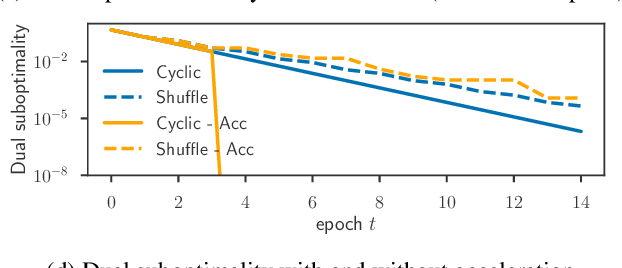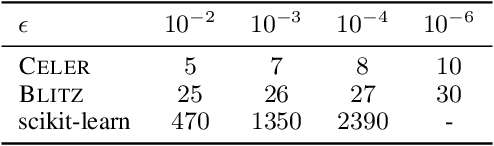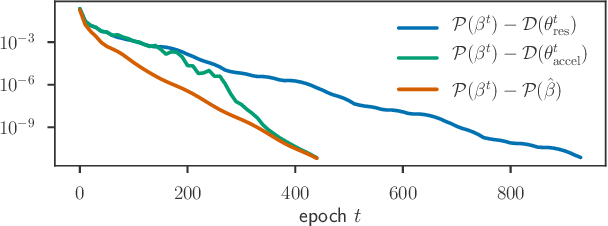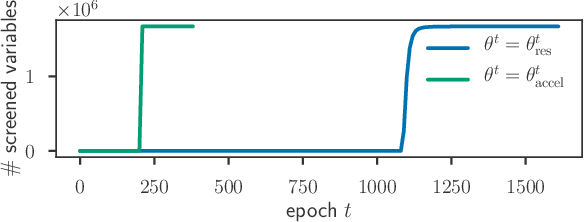Convex sparsity-inducing regularizations are ubiquitous in high-dimensional machine learning, but solving the resulting optimization problems can be slow. To accelerate solvers, state-of-the-art approaches consist in reducing the size of the optimization problem at hand. In the context of regression, this can be achieved either by discarding irrelevant features (screening techniques) or by prioritizing features likely to be included in the support of the solution (working set techniques). Duality comes into play at several steps in these techniques. Here, we propose an extrapolation technique starting from a sequence of iterates in the dual that leads to the construction of improved dual points. This enables a tighter control of optimality as used in stopping criterion, as well as better screening performance of Gap Safe rules. Finally, we propose a working set strategy based on an aggressive use of Gap Safe screening rules. Thanks to our new dual point construction, we show significant computational speedups on multiple real-world problems.

Click to Read Paper and Get Code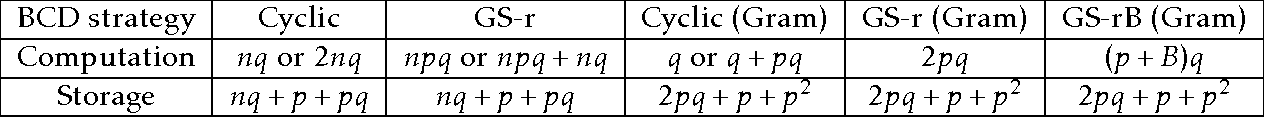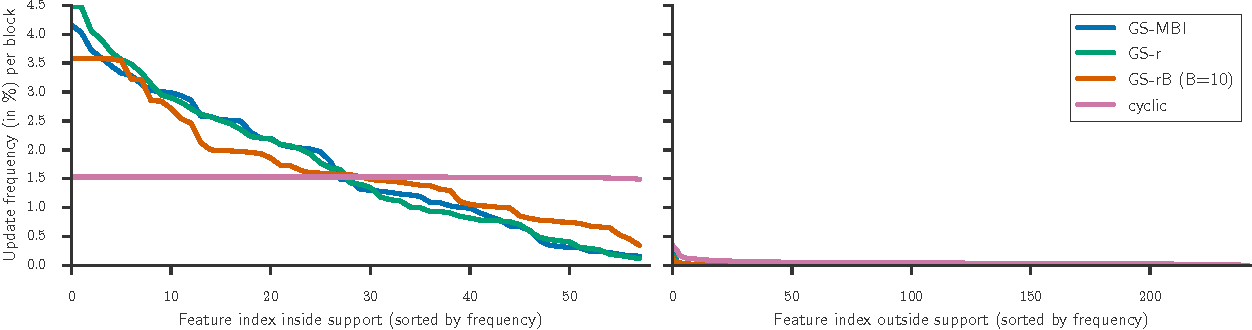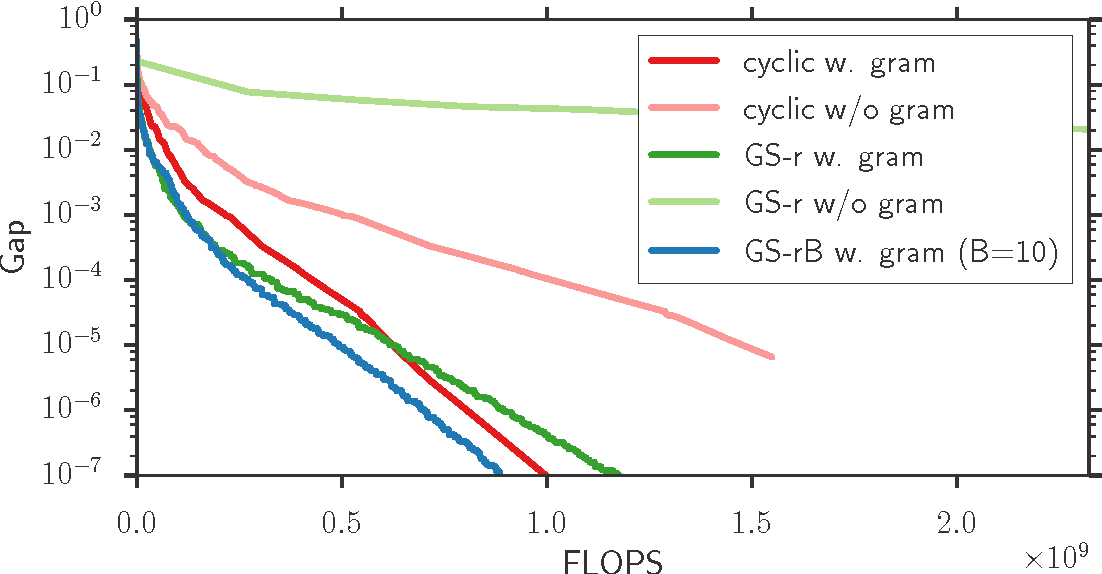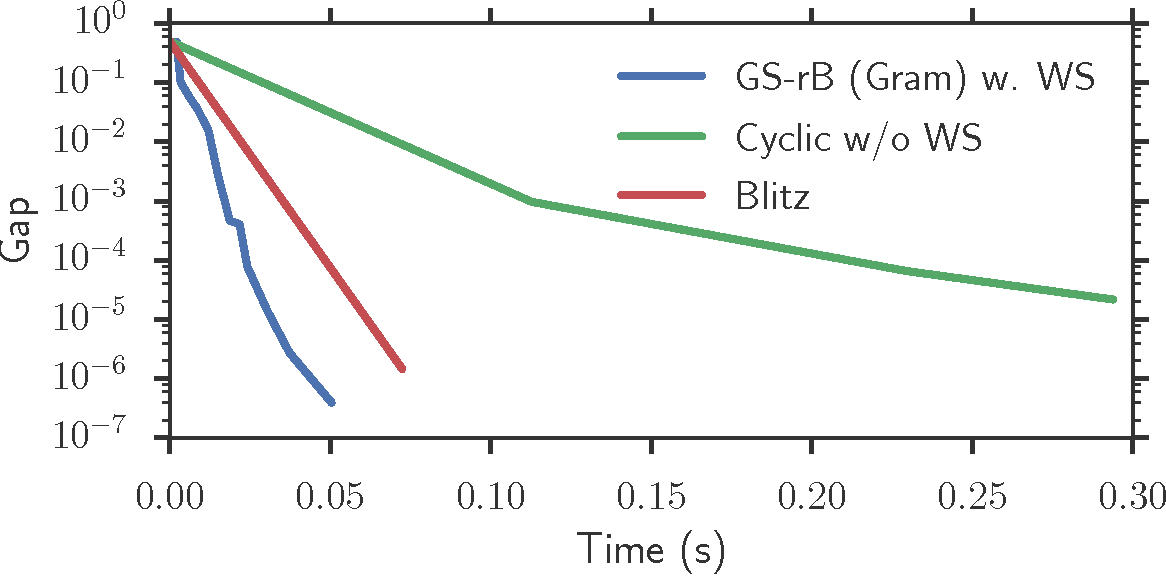Convex sparsity-promoting regularizations are ubiquitous in modern statistical learning. By construction, they yield solutions with few non-zero coefficients, which correspond to saturated constraints in the dual optimization formulation. Working set (WS) strategies are generic optimization techniques that consist in solving simpler problems that only consider a subset of constraints, whose indices form the WS. Working set methods therefore involve two nested iterations: the outer loop corresponds to the definition of the WS and the inner loop calls a solver for the subproblems. For the Lasso estimator a WS is a set of features, while for a Group Lasso it refers to a set of groups. In practice, WS are generally small in this context so the associated feature Gram matrix can fit in memory. Here we show that the Gauss-Southwell rule (a greedy strategy for block coordinate descent techniques) leads to fast solvers in this case. Combined with a working set strategy based on an aggressive use of so-called Gap Safe screening rules, we propose a solver achieving state-of-the-art performance on sparse learning problems. Results are presented on Lasso and multi-task Lasso estimators.

Click to Read Paper and Get Code
Screening rules allow to early discard irrelevant variables from the optimization in Lasso problems, or its derivatives, making solvers faster. In this paper, we propose new versions of the so-called $\textit{safe rules}$ for the Lasso. Based on duality gap considerations, our new rules create safe test regions whose diameters converge to zero, provided that one relies on a converging solver. This property helps screening out more variables, for a wider range of regularization parameter values. In addition to faster convergence, we prove that we correctly identify the active sets (supports) of the solutions in finite time. While our proposed strategy can cope with any solver, its performance is demonstrated using a coordinate descent algorithm particularly adapted to machine learning use cases. Significant computing time reductions are obtained with respect to previous safe rules.

* erratum to ICML 2015, "The authors would like to thanks Jalal Fadili and Jingwei Liang for helping clarifying some misleading statements on the equicorrelation set"
Click to Read Paper and Get Code
Recently it has been shown that the step sizes of a family of variance reduced gradient methods called the JacSketch methods depend on the expected smoothness constant. In particular, if this expected smoothness constant could be calculated a priori, then one could safely set much larger step sizes which would result in a much faster convergence rate. We fill in this gap, and provide simple closed form expressions for the expected smoothness constant and careful numerical experiments verifying these bounds. Using these bounds, and since the SAGA algorithm is part of this JacSketch family, we suggest a new standard practice for setting the step sizes and mini-batch size for SAGA that are competitive with a numerical grid search. Furthermore, we can now show that the total complexity of the SAGA algorithm decreases linearly in the mini-batch size up to a pre-defined value: the optimal mini-batch size. This is a rare result in the stochastic variance reduced literature, only previously shown for the Katyusha algorithm. Finally we conjecture that this is the case for many other stochastic variance reduced methods and that our bounds and analysis of the expected smoothness constant is key to extending these results.

* 34 pages, 27 figures
Click to Read Paper and Get Code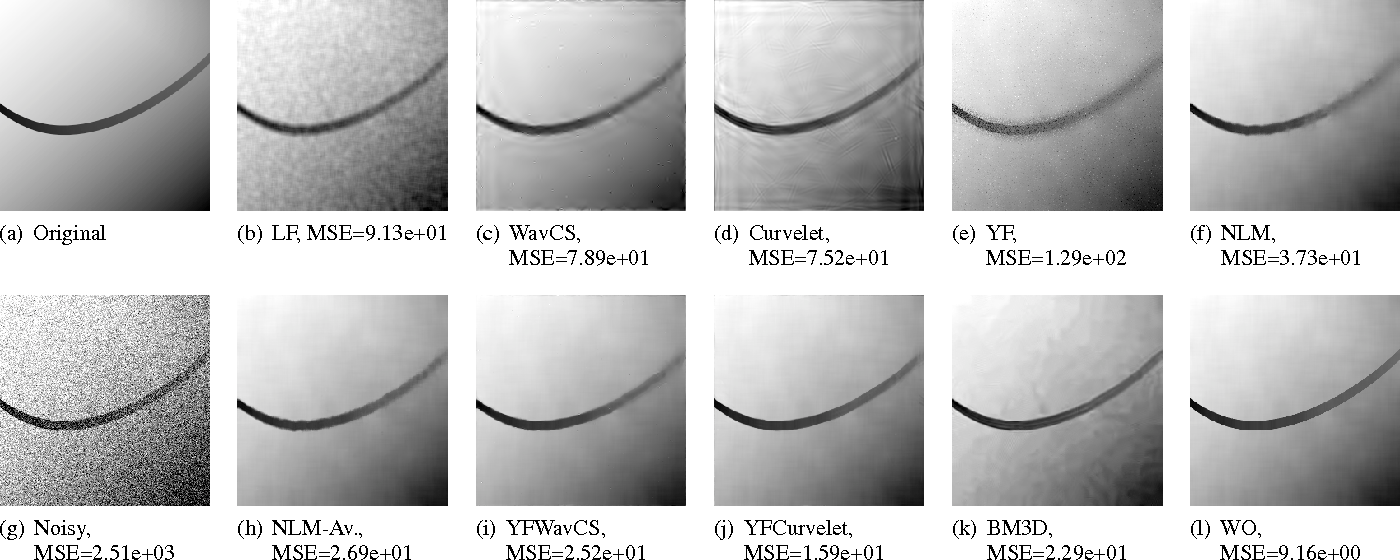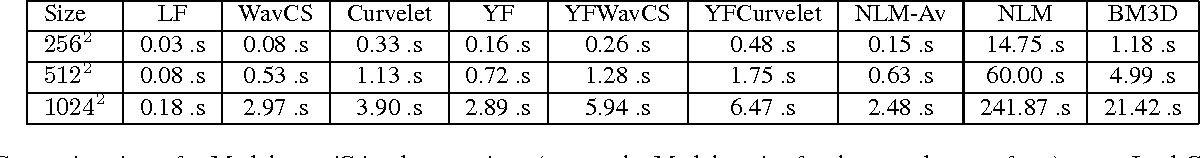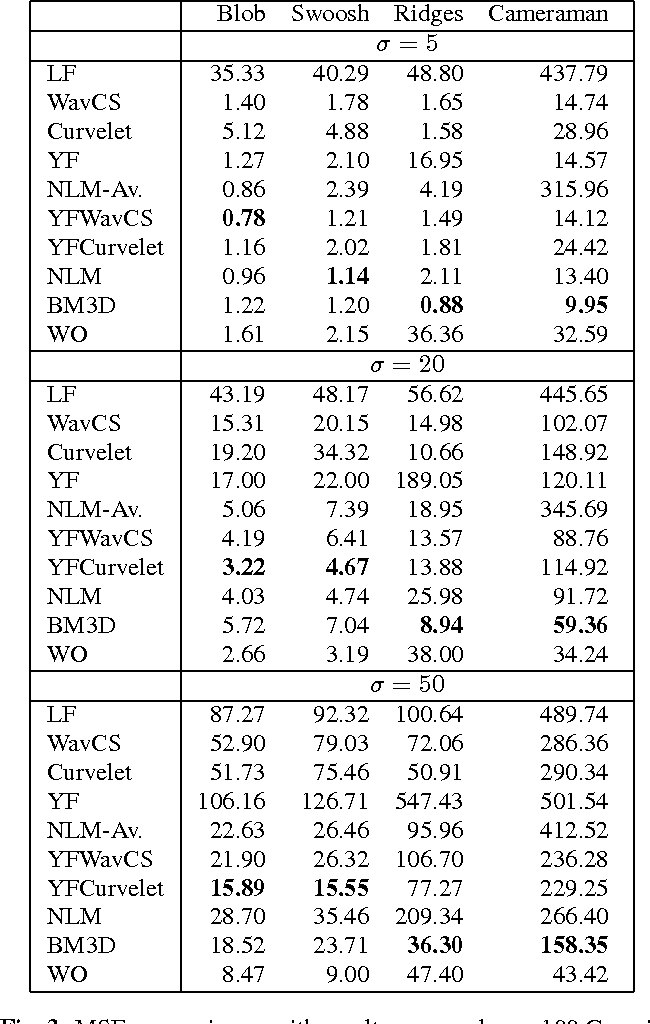This paper describes a simple image noise removal method which combines a preprocessing step with the Yaroslavsky filter for strong numerical, visual, and theoretical performance on a broad class of images. The framework developed is a two-stage approach. In the first stage the image is filtered with a classical denoising method (e.g., wavelet or curvelet thresholding). In the second stage a modification of the Yaroslavsky filter is performed on the original noisy image, where the weights of the filters are governed by pixel similarities in the denoised image from the first stage. Similar prefiltering ideas have proved effective previously in the literature, and this paper provides theoretical guarantees and important insight into why prefiltering can be effective. Empirically, this simple approach achieves very good performance for cartoon images, and can be computed much more quickly than current patch-based denoising algorithms.

Click to Read Paper and Get Code
This paper describes a novel theoretical characterization of the performance of non-local means (NLM) for noise removal. NLM has proven effective in a variety of empirical studies, but little is understood fundamentally about how it performs relative to classical methods based on wavelets or how various parameters (e.g., patch size) should be chosen. For cartoon images and images which may contain thin features and regular textures, the error decay rates of NLM are derived and compared with those of linear filtering, oracle estimators, variable-bandwidth kernel methods, Yaroslavsky's filter and wavelet thresholding estimators. The trade-off between global and local search for matching patches is examined, and the bias reduction associated with the local polynomial regression version of NLM is analyzed. The theoretical results are validated via simulations for 2D images corrupted by additive white Gaussian noise.

* 49 pages, 15 figures
Click to Read Paper and Get Code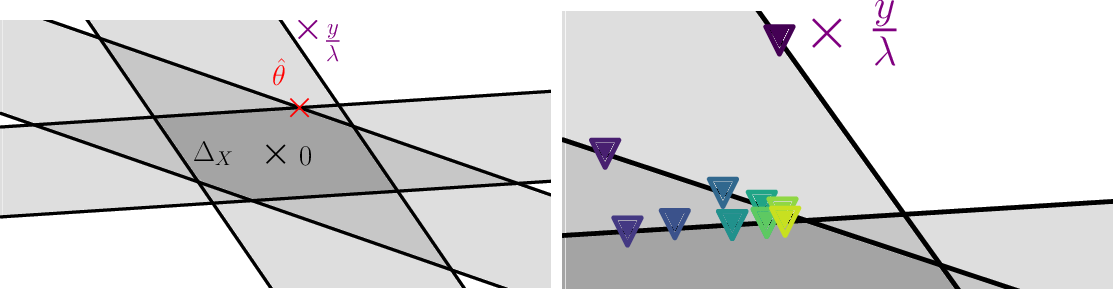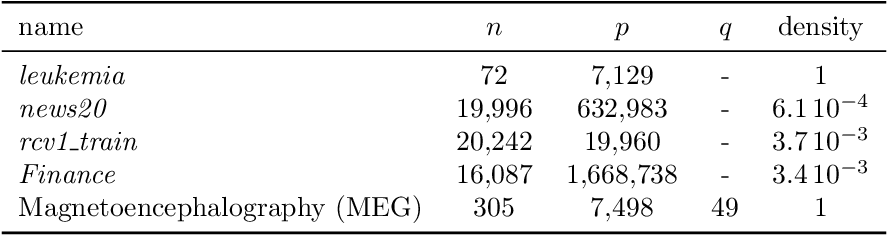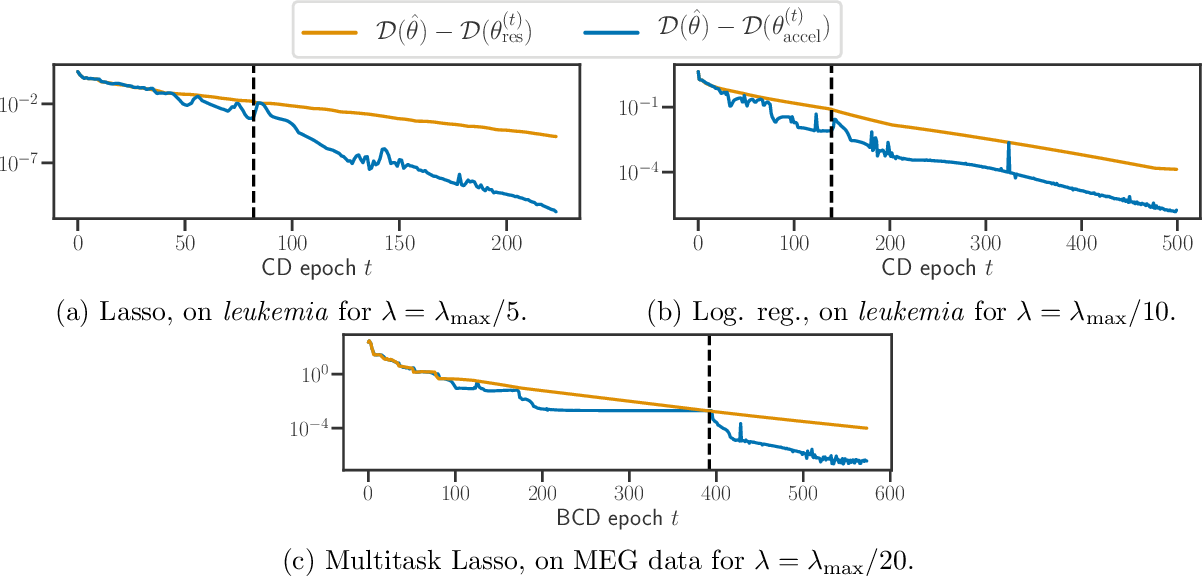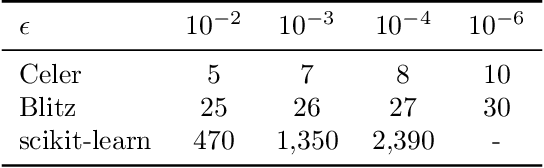Generalized Linear Models (GLM) form a wide class of regression and classification models, where prediction is a function of a linear combination of the input variables. For statistical inference in high dimension, sparsity inducing regularizations have proven to be useful while offering statistical guarantees. However, solving the resulting optimization problems can be challenging: even for popular iterative algorithms such as coordinate descent, one needs to loop over a large number of variables. To mitigate this, techniques known as screening rules and working sets diminish the size of the optimization problem at hand, either by progressively removing variables, or by solving a growing sequence of smaller problems. For both techniques, significant variables are identified thanks to convex duality arguments. In this paper, we show that the dual iterates of a GLM exhibit a Vector AutoRegressive (VAR) behavior after sign identification, when the primal problem is solved with proximal gradient descent or cyclic coordinate descent. Exploiting this regularity, one can construct dual points that offer tighter certificates of optimality, enhancing the performance of screening rules and helping to design competitive working set algorithms.

Click to Read Paper and Get Code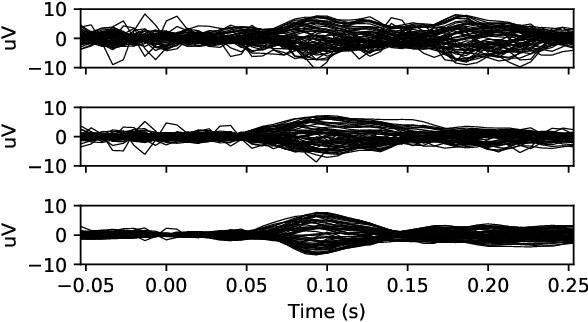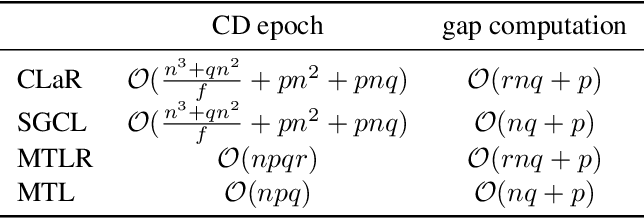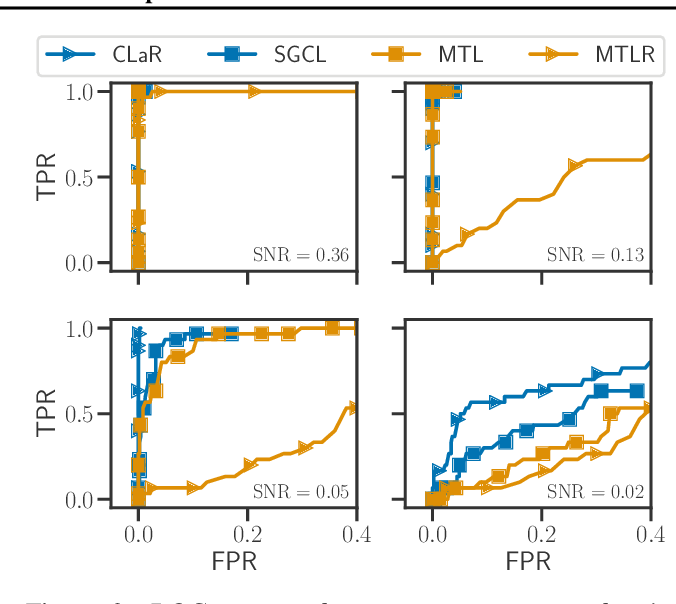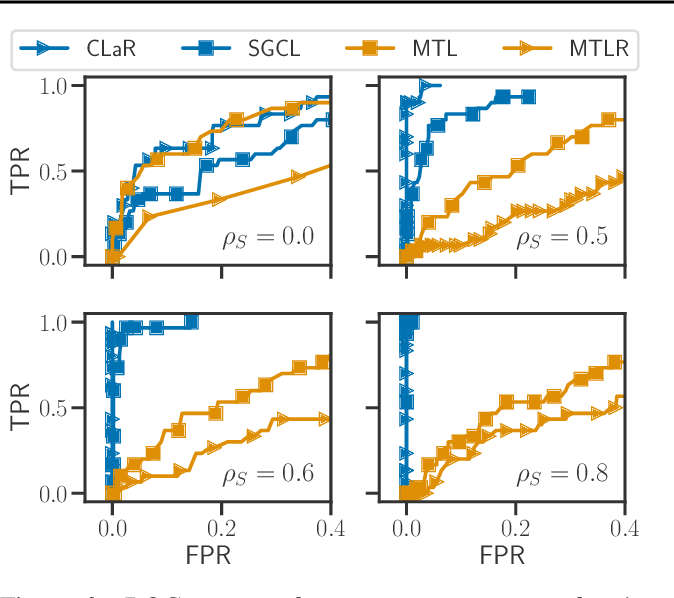Sparsity promoting norms are frequently used in high dimensional regression. A limitation of Lasso-type estimators is that the regulariza-tion parameter depends on the noise level which varies between datasets and experiments. Esti-mators such as the concomitant Lasso address this dependence by jointly estimating the noise level and the regression coefficients. As sample sizes are often limited in high dimensional regimes, simplified heteroscedastic models are customary. However, in many experimental applications , data is obtained by averaging multiple measurements. This helps reducing the noise variance, yet it dramatically reduces sample sizes, preventing refined noise modeling. In this work, we propose an estimator that can cope with complex heteroscedastic noise structures by using non-averaged measurements and a con-comitant formulation. The resulting optimization problem is convex, so thanks to smoothing theory, it is amenable to state-of-the-art proximal coordinate descent techniques that can leverage the expected sparsity of the solutions. Practical benefits are demonstrated on simulations and on neuroimaging applications.

Click to Read Paper and Get Code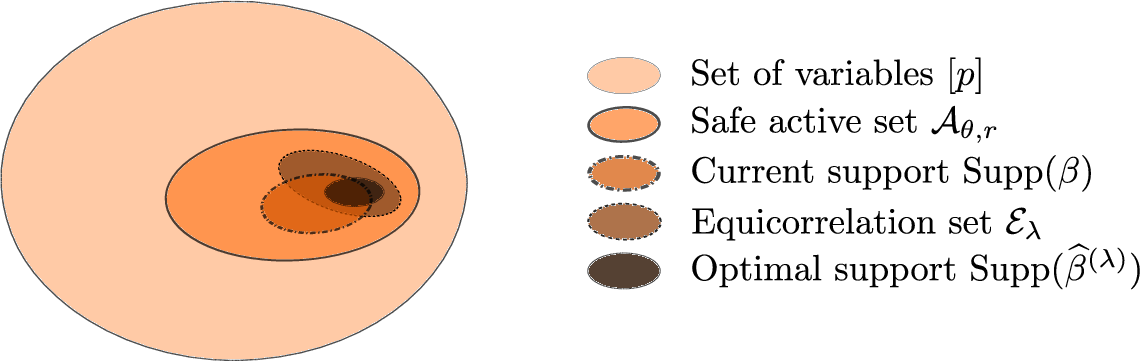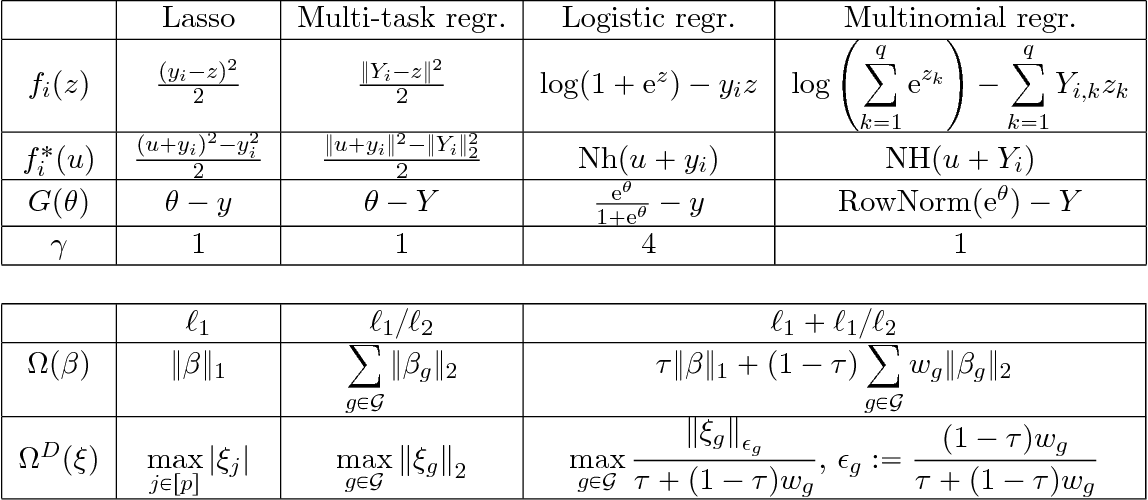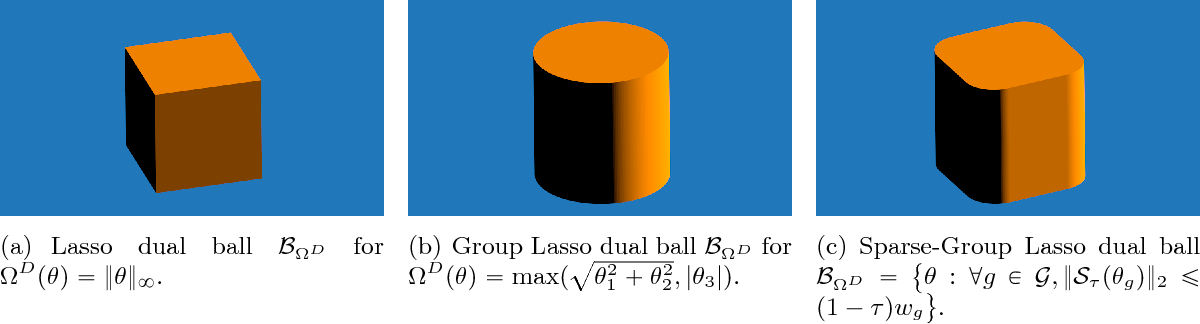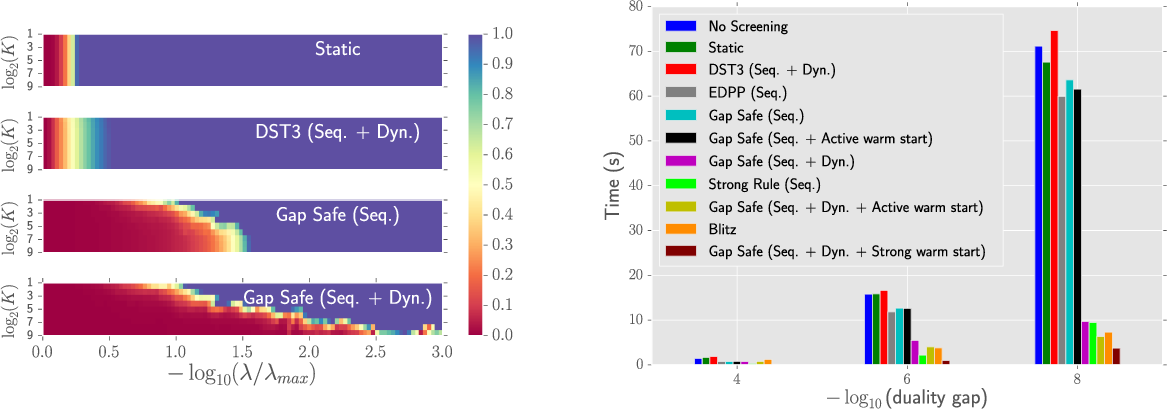In high dimensional regression settings, sparsity enforcing penalties have proved useful to regularize the data-fitting term. A recently introduced technique called screening rules propose to ignore some variables in the optimization leveraging the expected sparsity of the solutions and consequently leading to faster solvers. When the procedure is guaranteed not to discard variables wrongly the rules are said to be safe. In this work, we propose a unifying framework for generalized linear models regularized with standard sparsity enforcing penalties such as $\ell_1$ or $\ell_1/\ell_2$ norms. Our technique allows to discard safely more variables than previously considered safe rules, particularly for low regularization parameters. Our proposed Gap Safe rules (so called because they rely on duality gap computation) can cope with any iterative solver but are particularly well suited to (block) coordinate descent methods. Applied to many standard learning tasks, Lasso, Sparse-Group Lasso, multi-task Lasso, binary and multinomial logistic regression, etc., we report significant speed-ups compared to previously proposed safe rules on all tested data sets.

Click to Read Paper and Get Code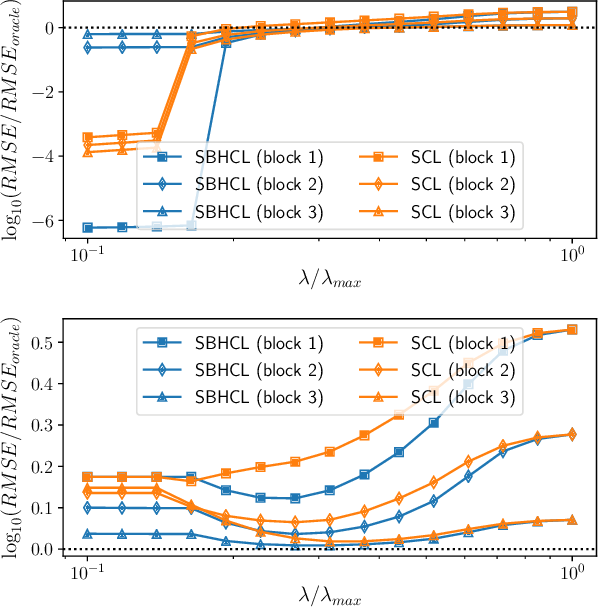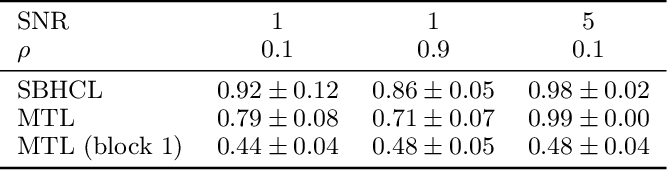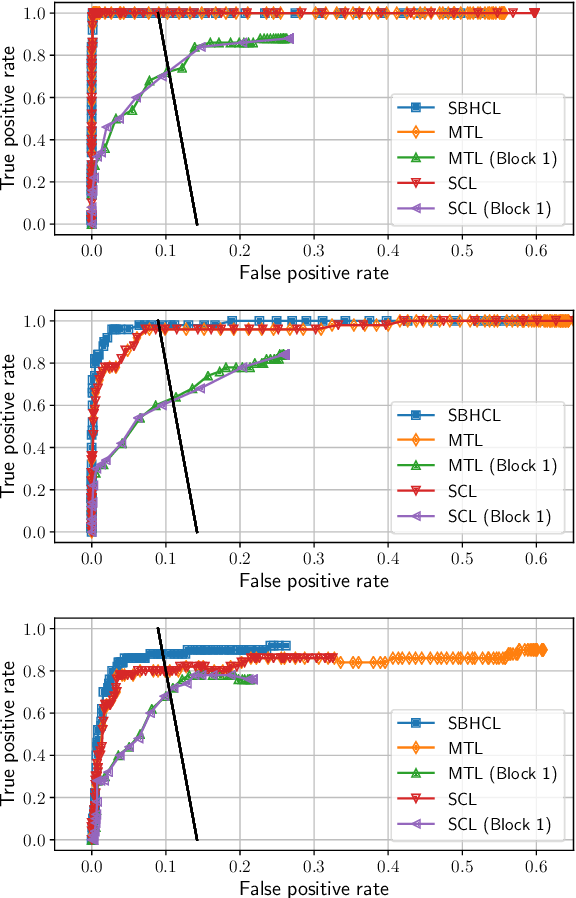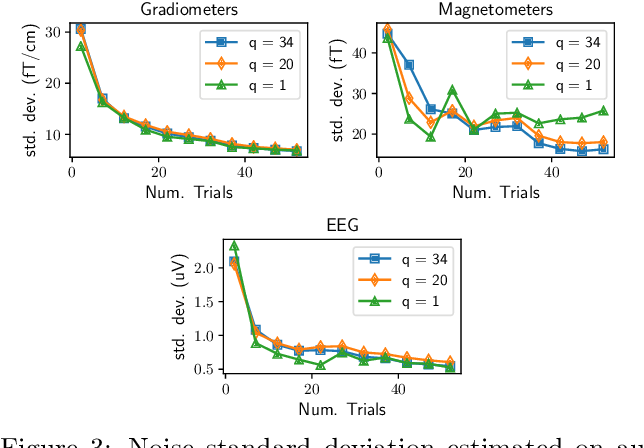In high dimension, it is customary to consider Lasso-type estimators to enforce sparsity. For standard Lasso theory to hold, the regularization parameter should be proportional to the noise level, yet the latter is generally unknown in practice. A possible remedy is to consider estimators, such as the Concomitant/Scaled Lasso, which jointly optimize over the regression coefficients as well as over the noise level, making the choice of the regularization independent of the noise level. However, when data from different sources are pooled to increase sample size, or when dealing with multimodal datasets, noise levels typically differ and new dedicated estimators are needed. In this work we provide new statistical and computational solutions to deal with such heteroscedastic regression models, with an emphasis on functional brain imaging with combined magneto- and electroencephalographic (M/EEG) signals. Adopting the formulation of Concomitant Lasso-type estimators, we propose a jointly convex formulation to estimate both the regression coefficients and the (square root of the) noise covariance. When our framework is instantiated to de-correlated noise, it leads to an efficient algorithm whose computational cost is not higher than for the Lasso and Concomitant Lasso, while addressing more complex noise structures. Numerical experiments demonstrate that our estimator yields improved prediction and support identification while correctly estimating the noise (square root) covariance. Results on multimodal neuroimaging problems with M/EEG data are also reported.

Click to Read Paper and Get Code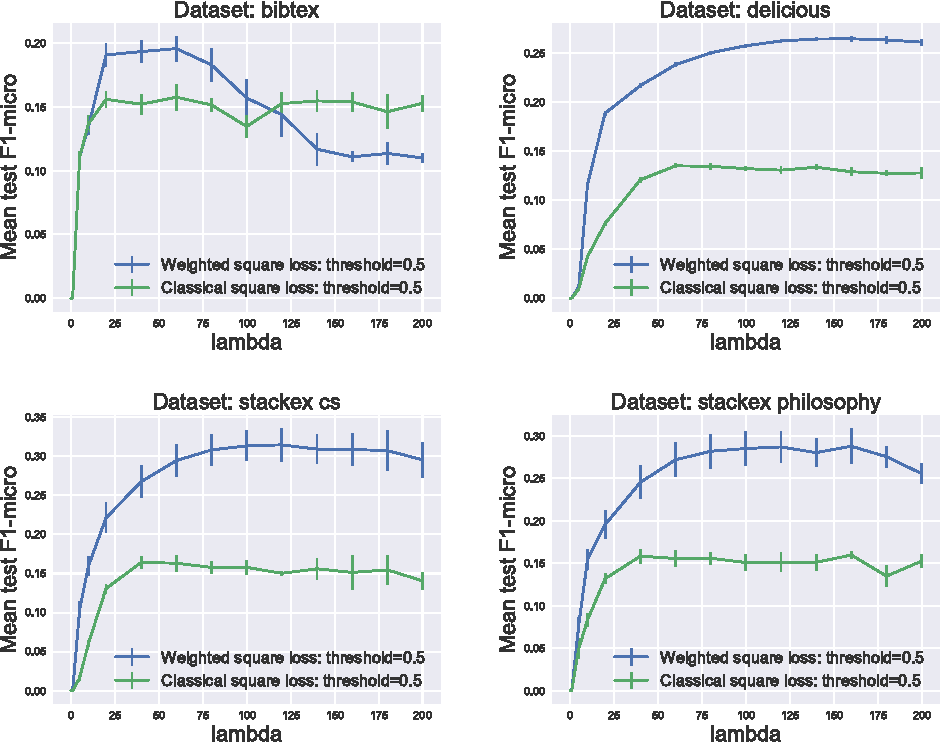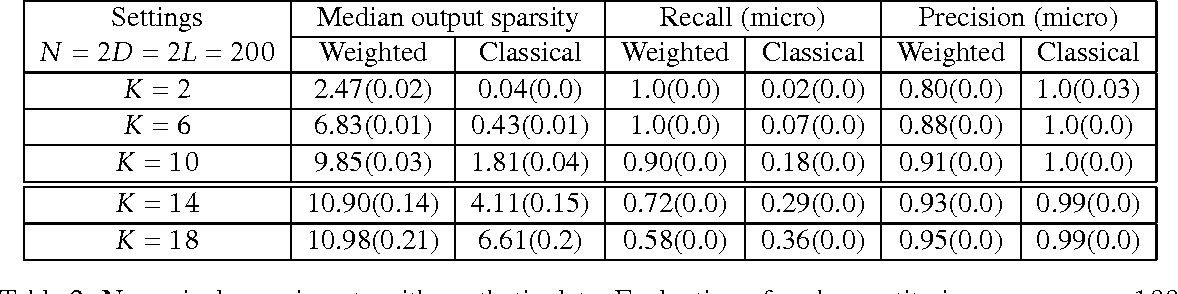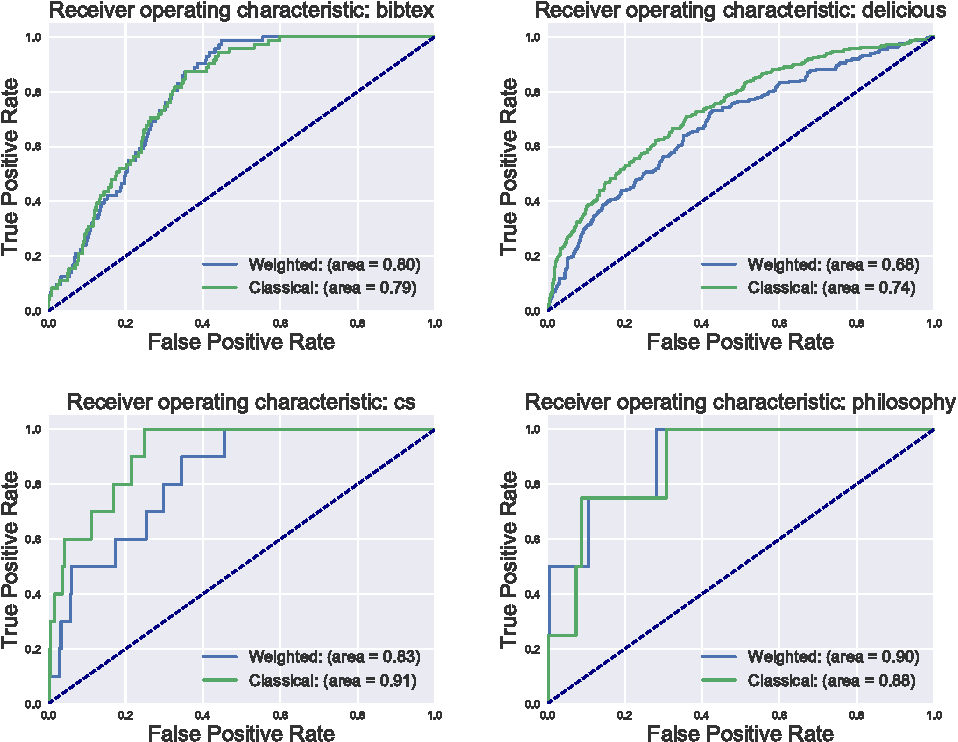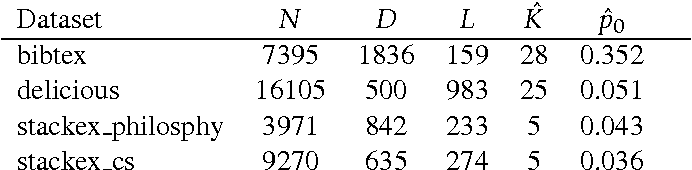The multi-label classification framework, where each observation can be associated with a set of labels, has generated a tremendous amount of attention over recent years. The modern multi-label problems are typically large-scale in terms of number of observations, features and labels, and the amount of labels can even be comparable with the amount of observations. In this context, different remedies have been proposed to overcome the curse of dimensionality. In this work, we aim at exploiting the output sparsity by introducing a new loss, called the sparse weighted Hamming loss. This proposed loss can be seen as a weighted version of classical ones, where active and inactive labels are weighted separately. Leveraging the influence of sparsity in the loss function, we provide improved generalization bounds for the empirical risk minimizer, a suitable property for large-scale problems. For this new loss, we derive rates of convergence linear in the underlying output-sparsity rather than linear in the number of labels. In practice, minimizing the associated risk can be performed efficiently by using convex surrogates and modern convex optimization algorithms. We provide experiments on various real-world datasets demonstrating the pertinence of our approach when compared to non-weighted techniques.

Click to Read Paper and Get Code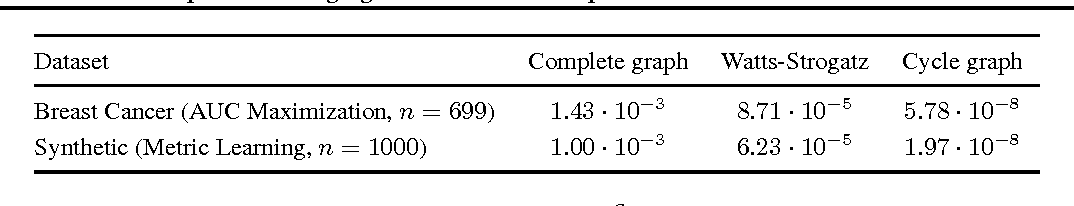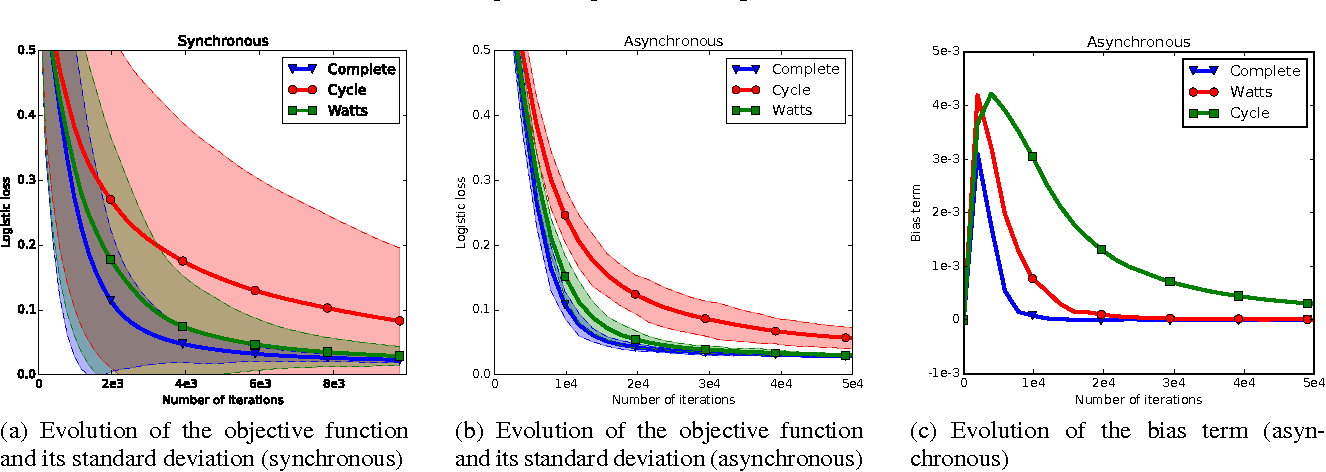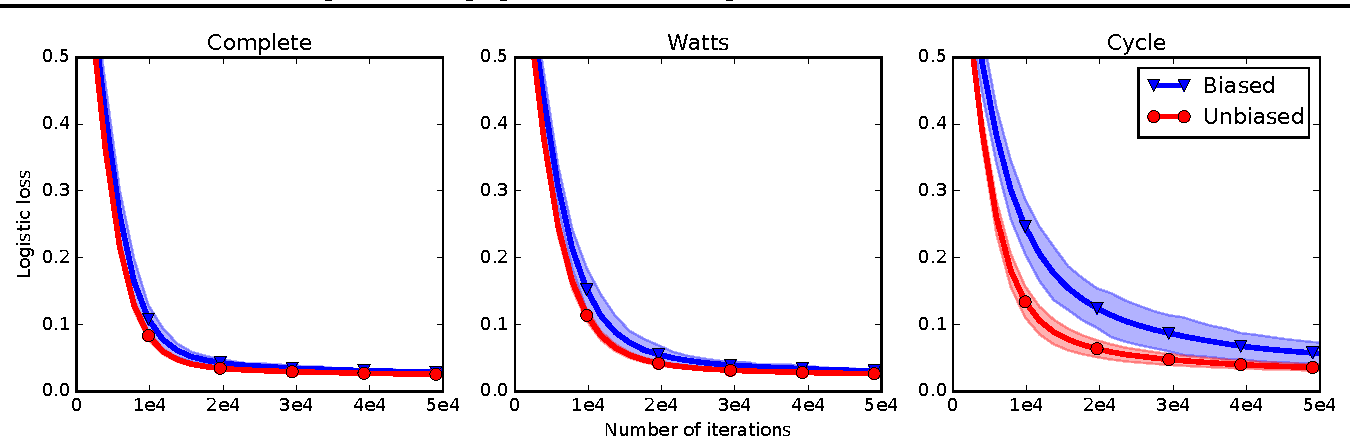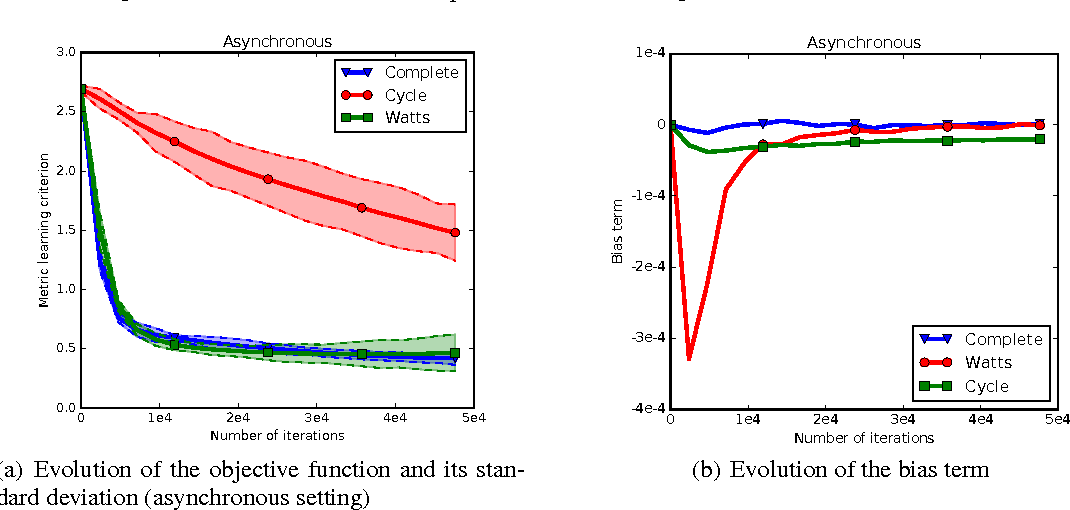In decentralized networks (of sensors, connected objects, etc.), there is an important need for efficient algorithms to optimize a global cost function, for instance to learn a global model from the local data collected by each computing unit. In this paper, we address the problem of decentralized minimization of pairwise functions of the data points, where these points are distributed over the nodes of a graph defining the communication topology of the network. This general problem finds applications in ranking, distance metric learning and graph inference, among others. We propose new gossip algorithms based on dual averaging which aims at solving such problems both in synchronous and asynchronous settings. The proposed framework is flexible enough to deal with constrained and regularized variants of the optimization problem. Our theoretical analysis reveals that the proposed algorithms preserve the convergence rate of centralized dual averaging up to an additive bias term. We present numerical simulations on Area Under the ROC Curve (AUC) maximization and metric learning problems which illustrate the practical interest of our approach.

Click to Read Paper and Get Code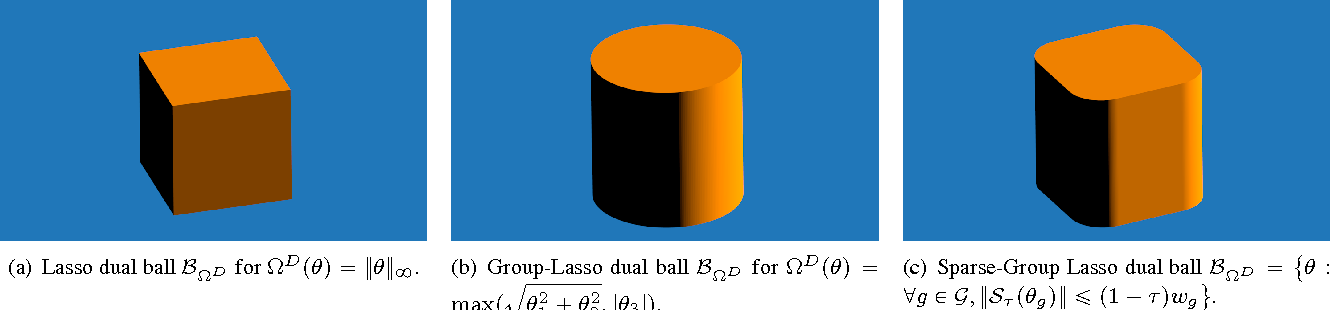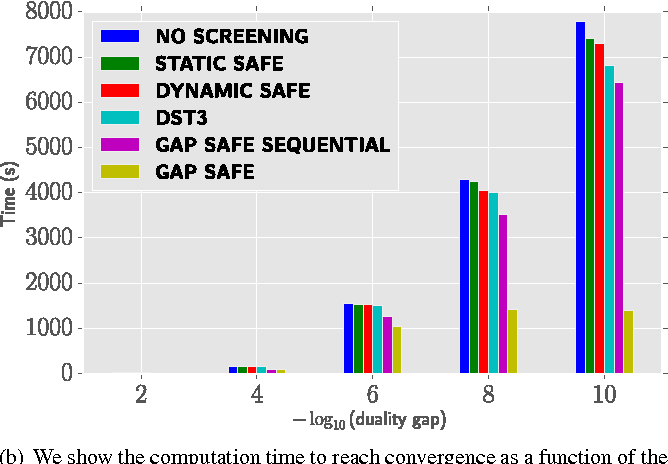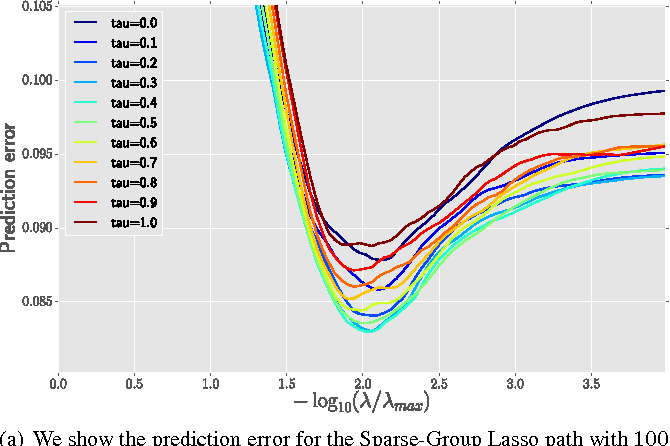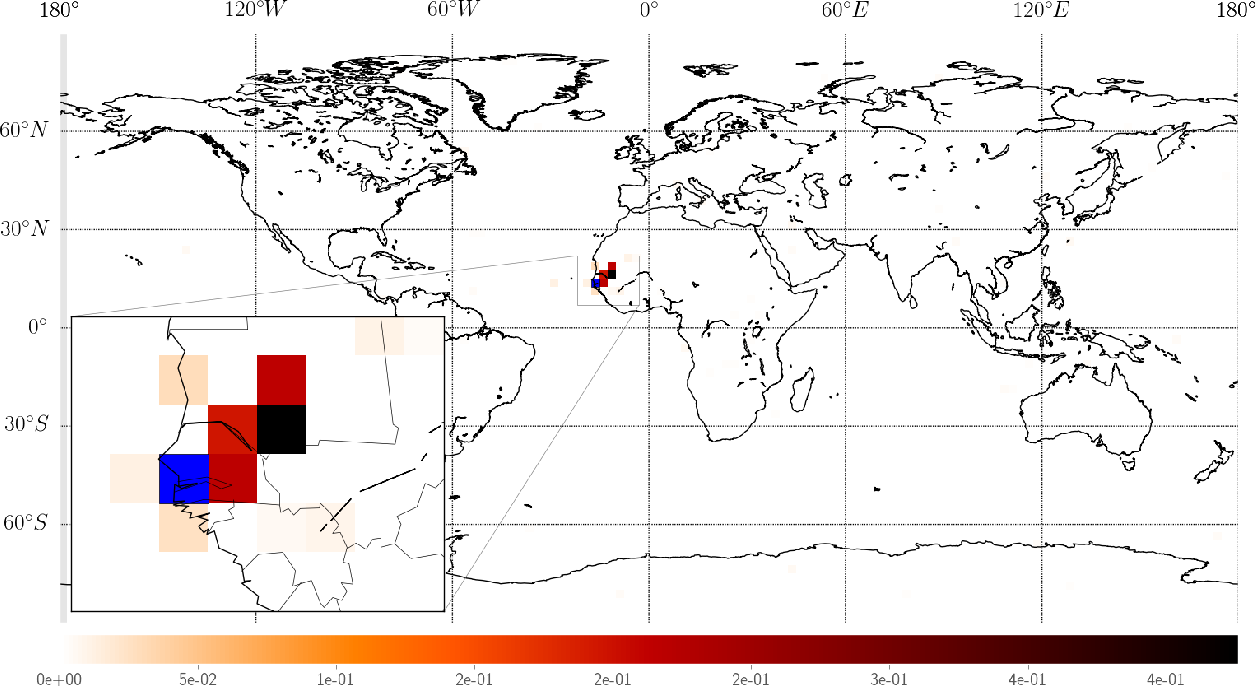In high dimensional settings, sparse structures are crucial for efficiency, either in term of memory, computation or performance. In some contexts, it is natural to handle more refined structures than pure sparsity, such as for instance group sparsity. Sparse-Group Lasso has recently been introduced in the context of linear regression to enforce sparsity both at the feature level and at the group level. We adapt to the case of Sparse-Group Lasso recent safe screening rules that discard early in the solver irrelevant features/groups. Such rules have led to important speed-ups for a wide range of iterative methods. Thanks to dual gap computations, we provide new safe screening rules for Sparse-Group Lasso and show significant gains in term of computing time for a coordinate descent implementation.

Click to Read Paper and Get Code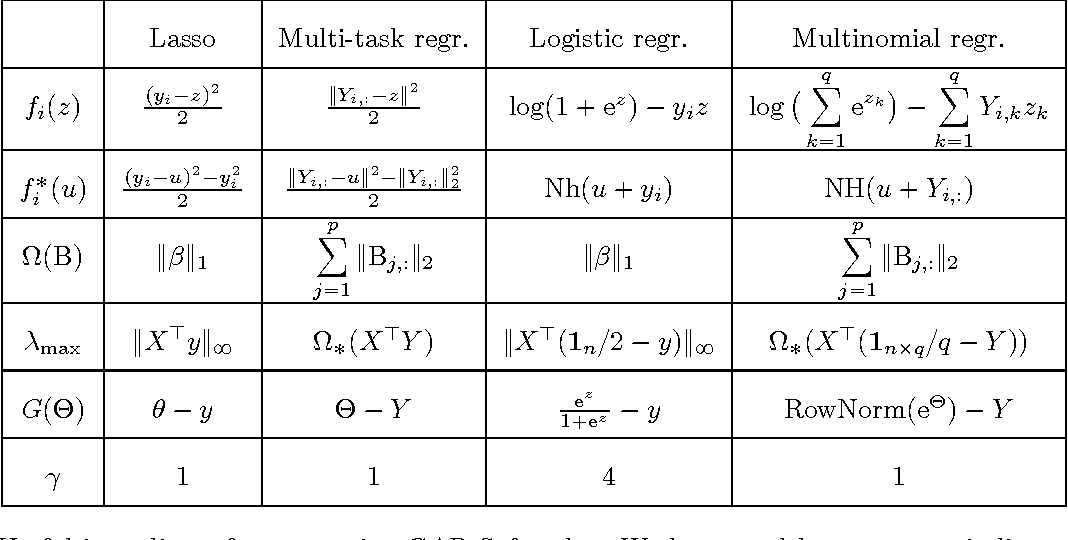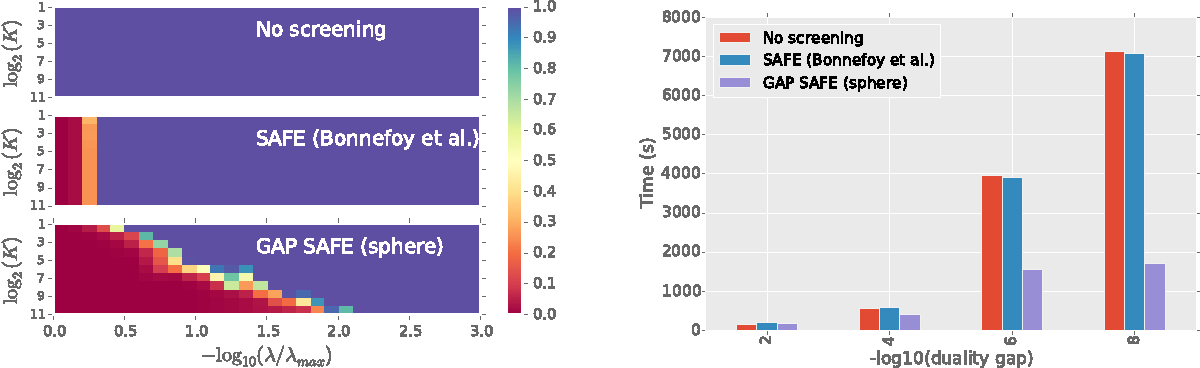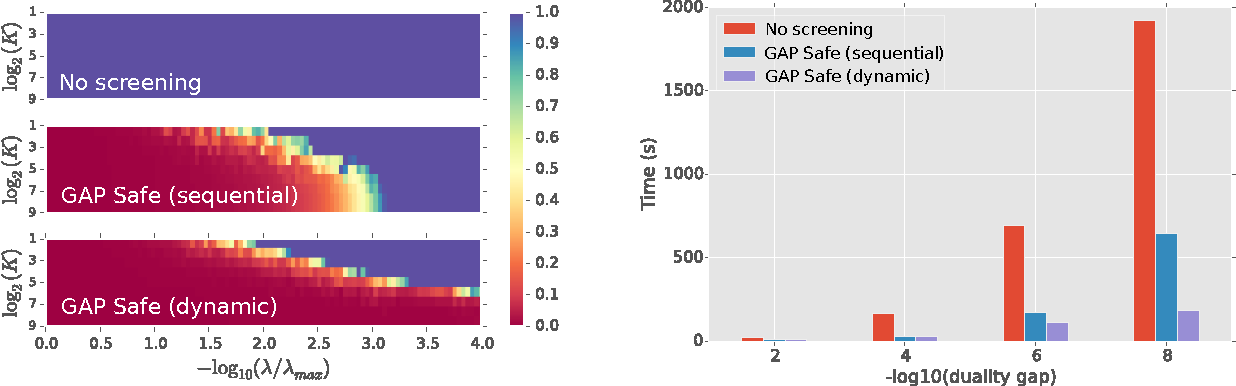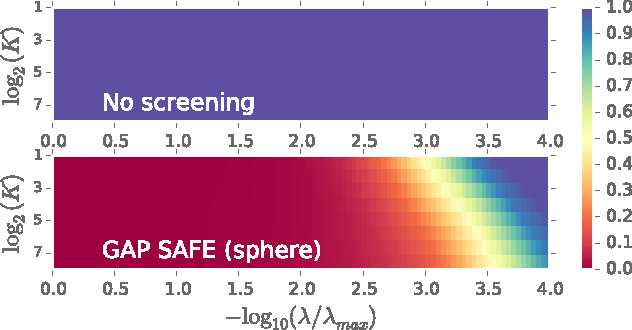High dimensional regression benefits from sparsity promoting regularizations. Screening rules leverage the known sparsity of the solution by ignoring some variables in the optimization, hence speeding up solvers. When the procedure is proven not to discard features wrongly the rules are said to be \emph{safe}. In this paper we derive new safe rules for generalized linear models regularized with $\ell_1$ and $\ell_1/\ell_2$ norms. The rules are based on duality gap computations and spherical safe regions whose diameters converge to zero. This allows to discard safely more variables, in particular for low regularization parameters. The GAP Safe rule can cope with any iterative solver and we illustrate its performance on coordinate descent for multi-task Lasso, binary and multinomial logistic regression, demonstrating significant speed ups on all tested datasets with respect to previous safe rules.

* in Proceedings of the 29-th Conference on Neural Information Processing Systems (NIPS), 2015
Click to Read Paper and Get Code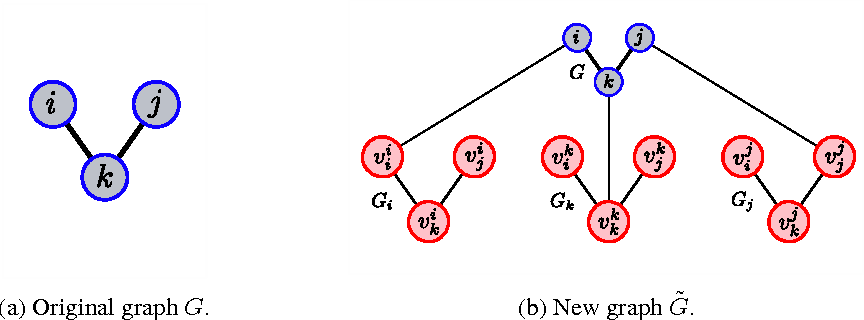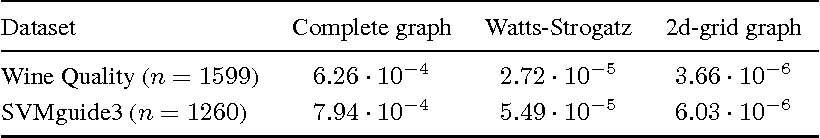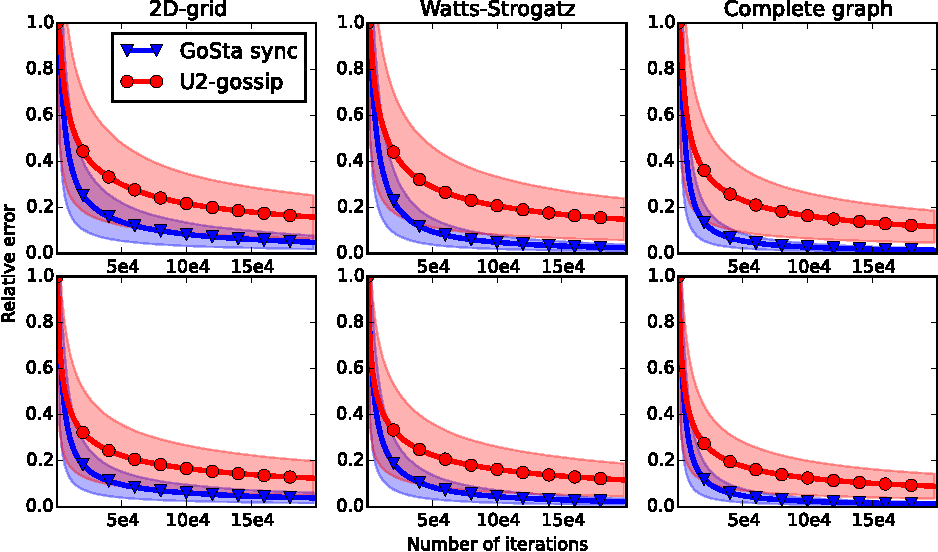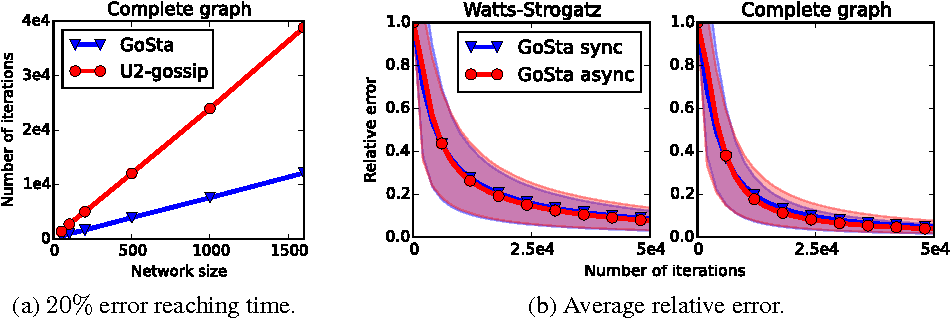Efficient and robust algorithms for decentralized estimation in networks are essential to many distributed systems. Whereas distributed estimation of sample mean statistics has been the subject of a good deal of attention, computation of $U$-statistics, relying on more expensive averaging over pairs of observations, is a less investigated area. Yet, such data functionals are essential to describe global properties of a statistical population, with important examples including Area Under the Curve, empirical variance, Gini mean difference and within-cluster point scatter. This paper proposes new synchronous and asynchronous randomized gossip algorithms which simultaneously propagate data across the network and maintain local estimates of the $U$-statistic of interest. We establish convergence rate bounds of $O(1/t)$ and $O(\log t / t)$ for the synchronous and asynchronous cases respectively, where $t$ is the number of iterations, with explicit data and network dependent terms. Beyond favorable comparisons in terms of rate analysis, numerical experiments provide empirical evidence the proposed algorithms surpasses the previously introduced approach.

* to be presented at NIPS 2015
Click to Read Paper and Get Code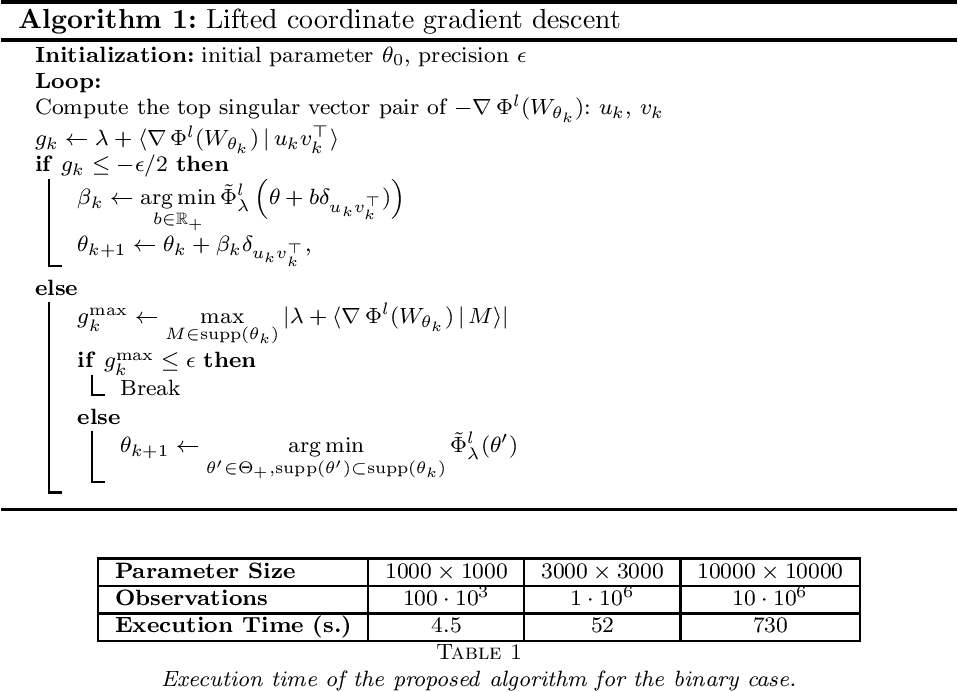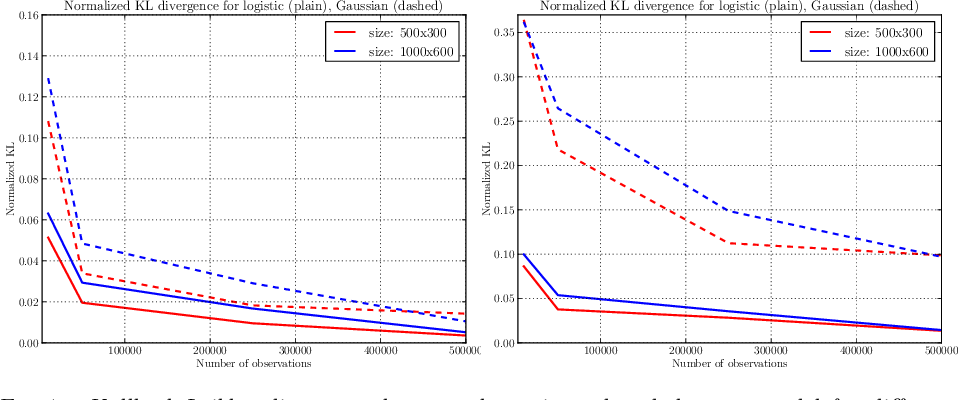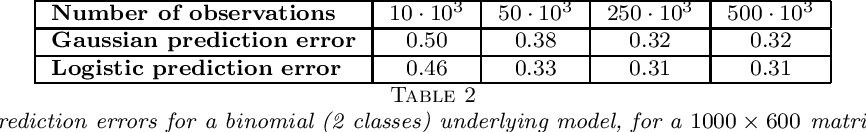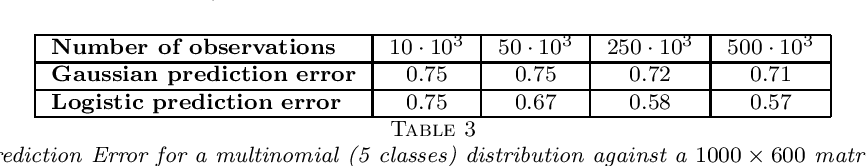The task of estimating a matrix given a sample of observed entries is known as the \emph{matrix completion problem}. Most works on matrix completion have focused on recovering an unknown real-valued low-rank matrix from a random sample of its entries. Here, we investigate the case of highly quantized observations when the measurements can take only a small number of values. These quantized outputs are generated according to a probability distribution parametrized by the unknown matrix of interest. This model corresponds, for example, to ratings in recommender systems or labels in multi-class classification. We consider a general, non-uniform, sampling scheme and give theoretical guarantees on the performance of a constrained, nuclear norm penalized maximum likelihood estimator. One important advantage of this estimator is that it does not require knowledge of the rank or an upper bound on the nuclear norm of the unknown matrix and, thus, it is adaptive. We provide lower bounds showing that our estimator is minimax optimal. An efficient algorithm based on lifted coordinate gradient descent is proposed to compute the estimator. A limited Monte-Carlo experiment, using both simulated and real data is provided to support our claims.

Click to Read Paper and Get Code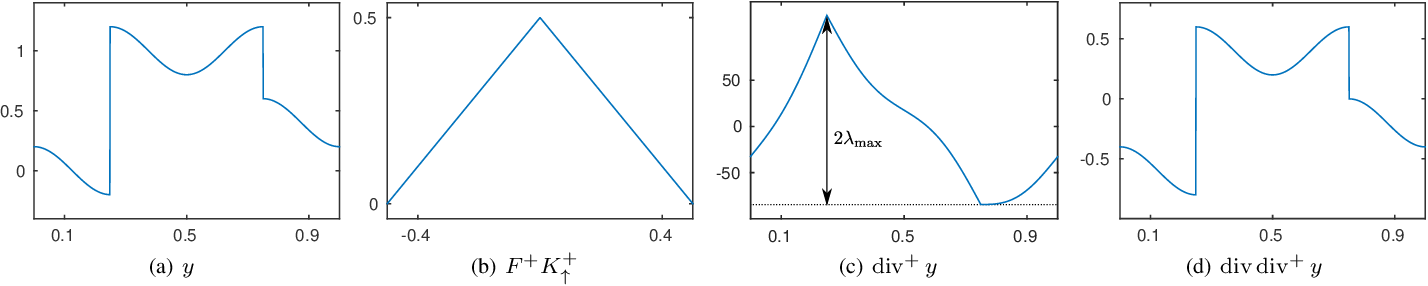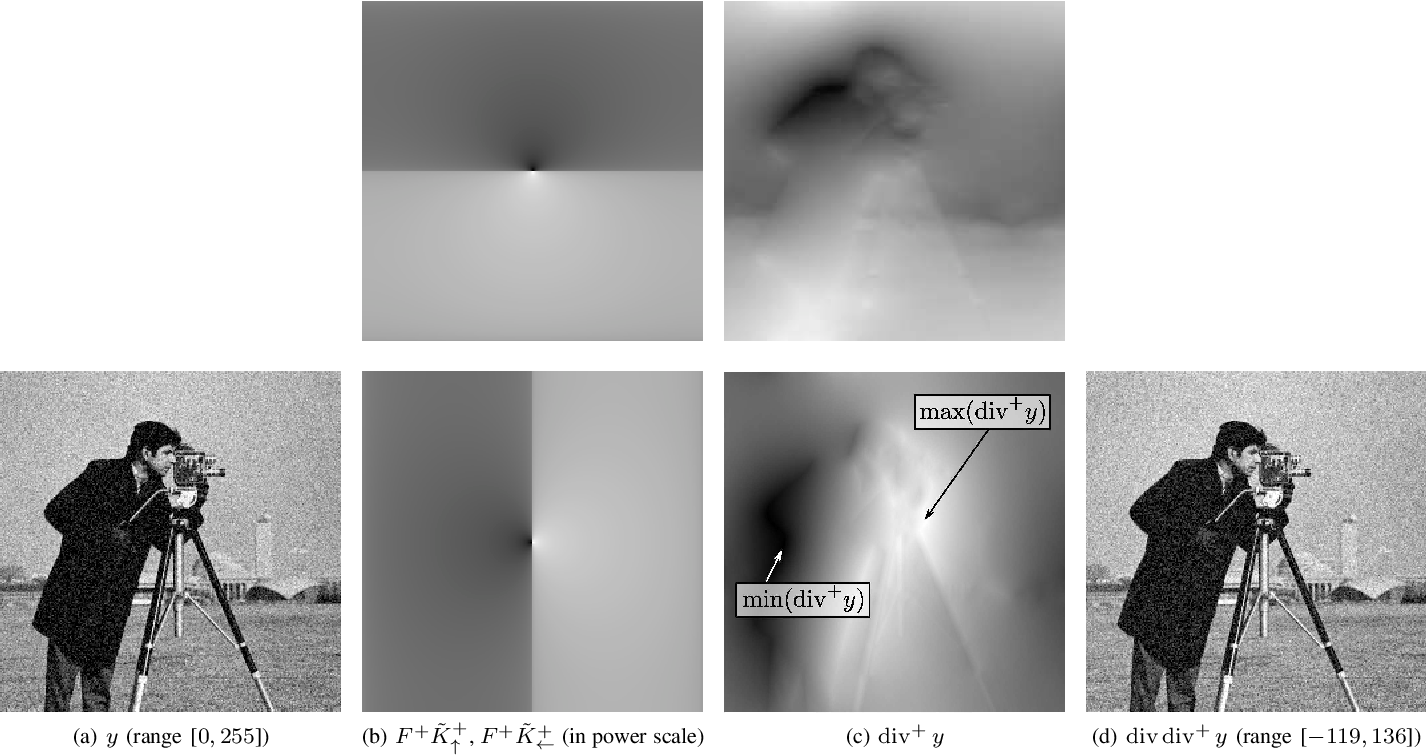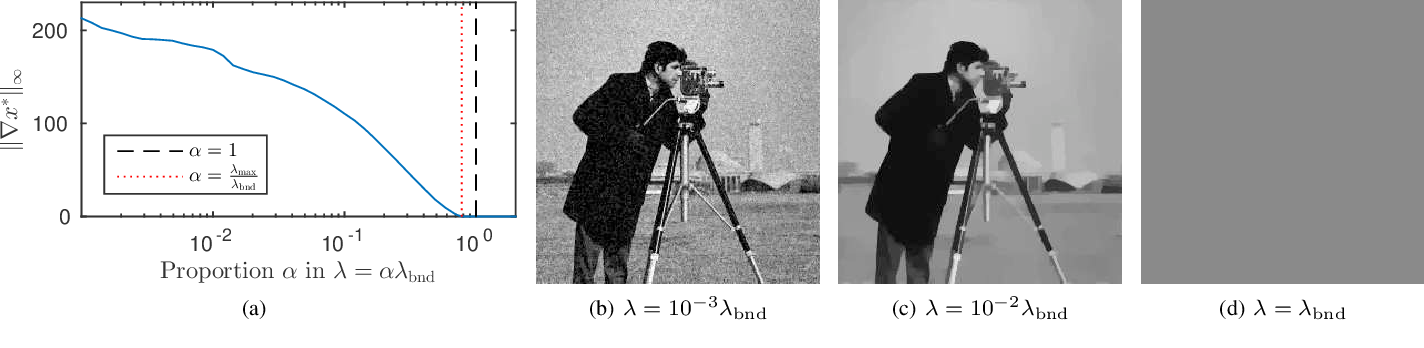We focus on the maximum regularization parameter for anisotropic total-variation denoising. It corresponds to the minimum value of the regularization parameter above which the solution remains constant. While this value is well know for the Lasso, such a critical value has not been investigated in details for the total-variation. Though, it is of importance when tuning the regularization parameter as it allows fixing an upper-bound on the grid for which the optimal parameter is sought. We establish a closed form expression for the one-dimensional case, as well as an upper-bound for the two-dimensional case, that appears reasonably tight in practice. This problem is directly linked to the computation of the pseudo-inverse of the divergence, which can be quickly obtained by performing convolutions in the Fourier domain.

Click to Read Paper and Get Code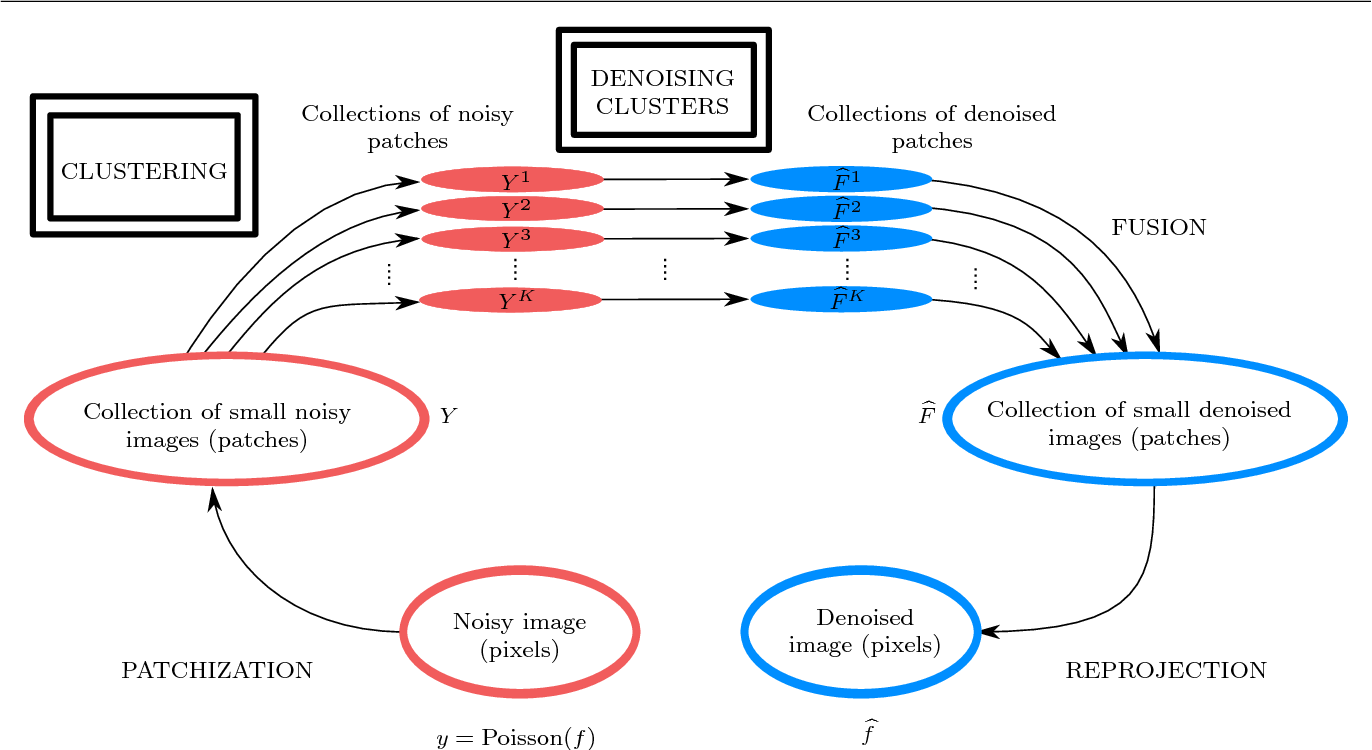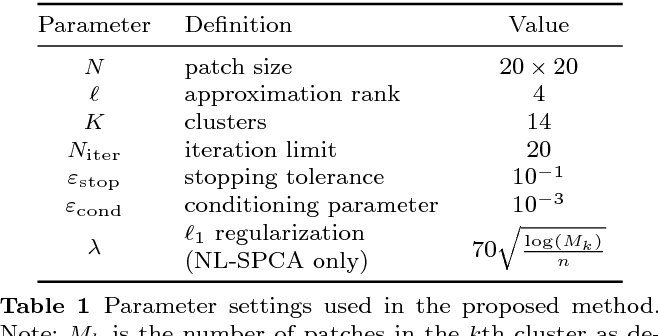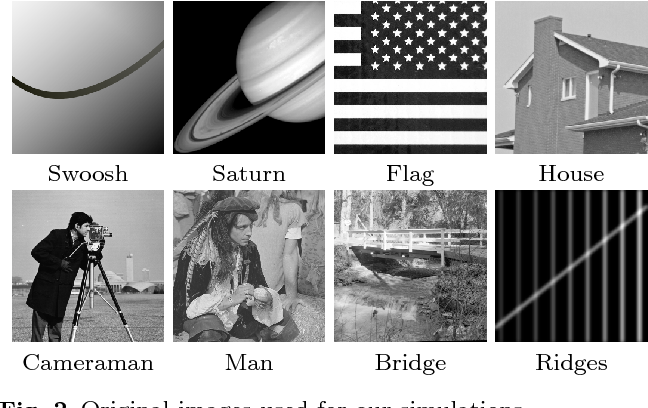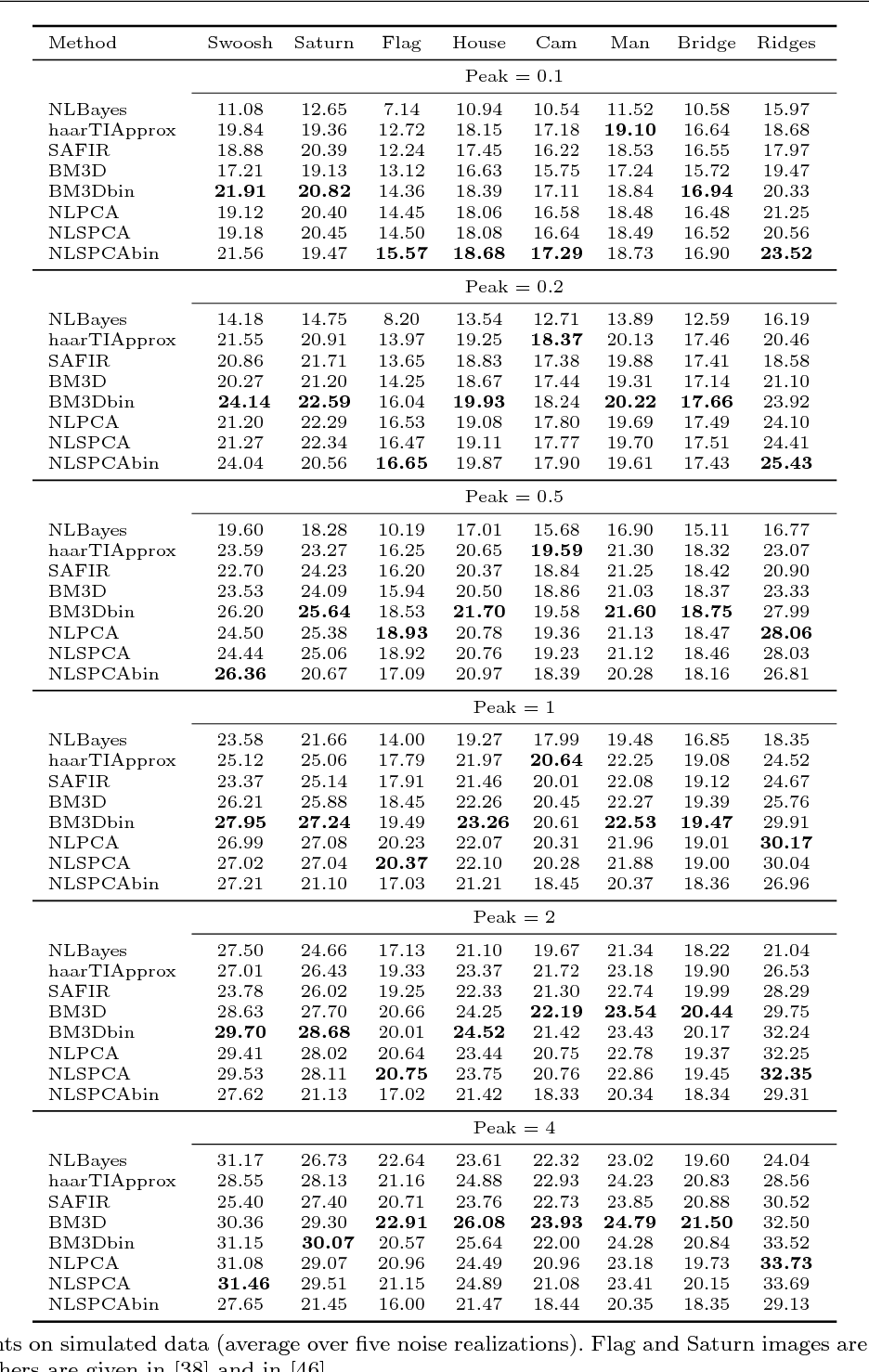Photon-limited imaging arises when the number of photons collected by a sensor array is small relative to the number of detector elements. Photon limitations are an important concern for many applications such as spectral imaging, night vision, nuclear medicine, and astronomy. Typically a Poisson distribution is used to model these observations, and the inherent heteroscedasticity of the data combined with standard noise removal methods yields significant artifacts. This paper introduces a novel denoising algorithm for photon-limited images which combines elements of dictionary learning and sparse patch-based representations of images. The method employs both an adaptation of Principal Component Analysis (PCA) for Poisson noise and recently developed sparsity-regularized convex optimization algorithms for photon-limited images. A comprehensive empirical evaluation of the proposed method helps characterize the performance of this approach relative to other state-of-the-art denoising methods. The results reveal that, despite its conceptual simplicity, Poisson PCA-based denoising appears to be highly competitive in very low light regimes.

* erratum: Image man is wrongly name pepper in the journal version
Click to Read Paper and Get Code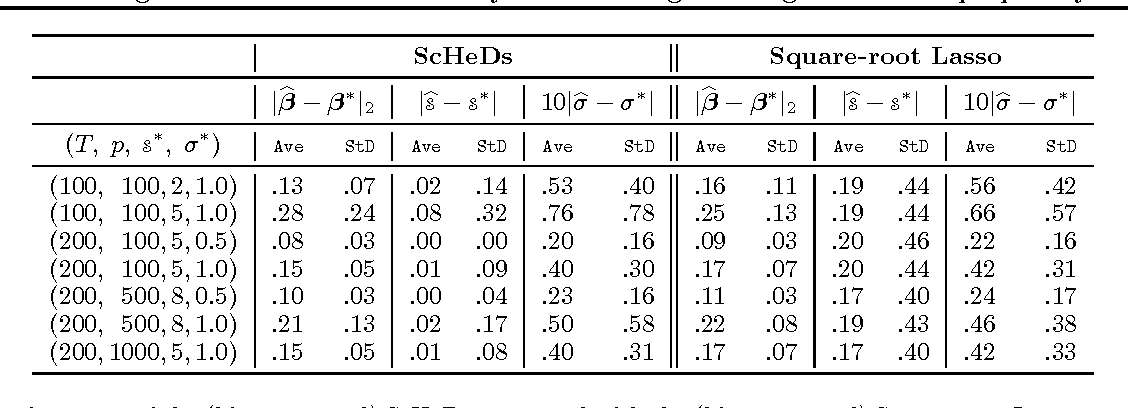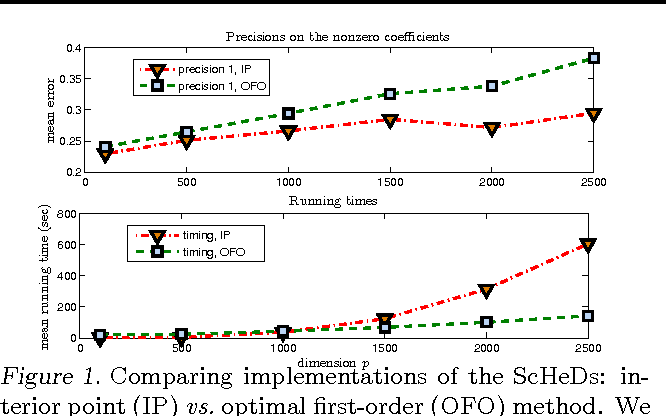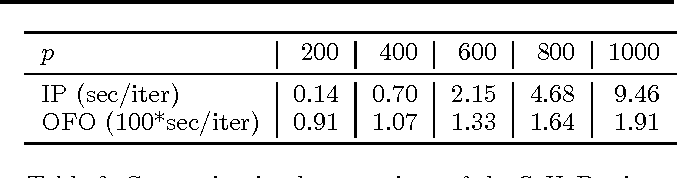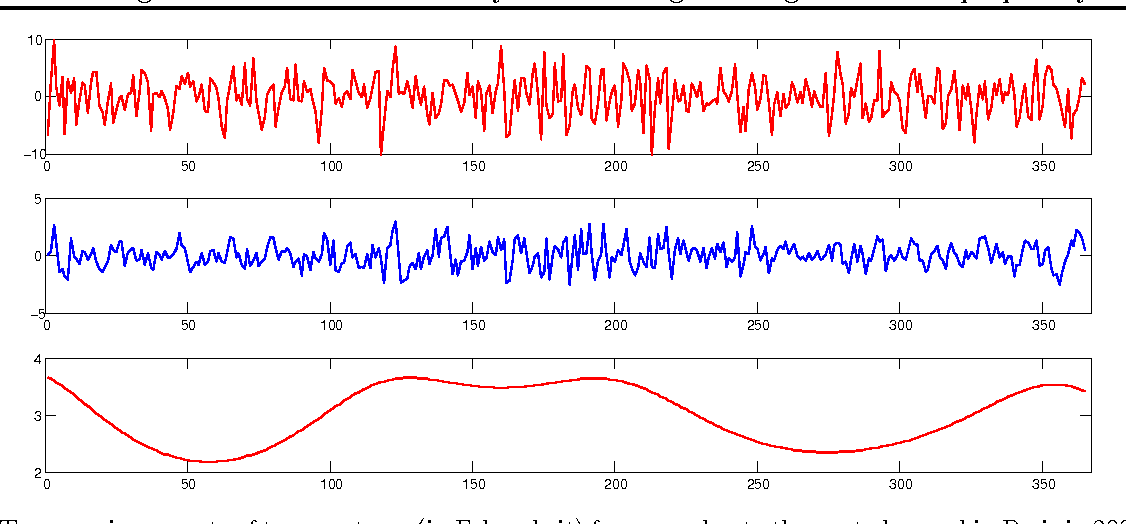Popular sparse estimation methods based on $\ell_1$-relaxation, such as the Lasso and the Dantzig selector, require the knowledge of the variance of the noise in order to properly tune the regularization parameter. This constitutes a major obstacle in applying these methods in several frameworks---such as time series, random fields, inverse problems---for which the noise is rarely homoscedastic and its level is hard to know in advance. In this paper, we propose a new approach to the joint estimation of the conditional mean and the conditional variance in a high-dimensional (auto-) regression setting. An attractive feature of the proposed estimator is that it is efficiently computable even for very large scale problems by solving a second-order cone program (SOCP). We present theoretical analysis and numerical results assessing the performance of the proposed procedure.

* Proceedings of the 30 th International Conference on Machine Learning (2013) http://icml.cc/2013/?page_id=43
Click to Read Paper and Get Code